AJP Excel Information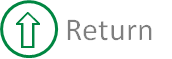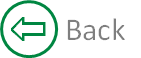##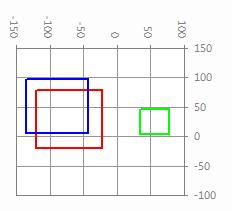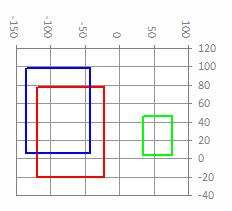##### Scatter plots in Excel show data that has no intrinsic spatial component; e.g., time series of cash flows for financial applications, waveforms for engineering applications, etc. Left to its own devices, Excel sets chart scales automatically, adjusting them generally to maximize the area of the plot that contains the data within the overall plot area -- and generally does it pretty well. Sometimes, though, we use scatter plots where the x and y axes represent true linear dimensions, and in those cases, Excel's default behavior doesn't help at all. We'd like equal spans (the size of max - min on each axis) to be the same, so that squares, for example, are actually square. A sub in the attached workbook does that, and shows a simple example that compares what the sub does to what Excel does by default. In your own application, you (the user) first create a chart that has equal height and width for the plot area. Then as data changes, you pass the min and max x and y values to the sub and it adjusts the scales to have equal spans and the major unit to be common to both axes. In the example, the major unit is a fixed size, but you could compute it based on the data if you wish. There's another (and more practical) example at http://www.excelforum.com/excel-general/672617-calculating-the-radius-of-a-curve.html

Function SetSquareAxes(cht As Chart, dBuf As Double, dInc As Double, _
ByVal xMin As Double, ByVal xMax As Double, _
ByVal yMin As Double, ByVal yMax As Double) As Boolean
' shg 2009-0220

' Sets the chart scales to
'   o   be of equal span
'   o   start and end on a multiple of dInc, and have dInc as the major unit
'   o   contain all points with a minimum buffer distance of dBuf to the edges
'   o   center the points in the plot area within the constraints above

' E.g.,

'    SetSquareAxes Sheet1.ChartObjects(1).Chart, 100, 500, _
'                  WorksheetFunction.Min(rngX.Value), _
'                  WorksheetFunction.Max(rngX.Value), _
'                  WorksheetFunction.Min(rngY.Value), _
'                  WorksheetFunction.Max(rngY.Value)

' Returns True if successful

Static WF   As WorksheetFunction
Dim xCtr    As Double
Dim yCtr    As Double
Dim dRad    As Double   ' half-dimension of bounding box
Dim dDelta  As Double   ' common span of x and y scales

' verify cht is a scatterchart
Select Case cht.SeriesCollection(1).ChartType
Case xlXYScatter, xlXYScatterLines, xlXYScatterSmooth, _
xlXYScatterLinesNoMarkers, xlXYScatterSmoothNoMarkers
' all good ...
Case Else
MsgBox "Chart type must be XY (Scatter)", vbOKOnly, "SetSquareAxes"
Exit Function
End Select

If WF Is Nothing Then Set WF = WorksheetFunction

' compute center and bounding box radius
xCtr = (xMax + xMin) / 2#
yCtr = (yMax + yMin) / 2#
dRad = WF.Max(xMax - xCtr, yMax - yCtr) + dBuf

' compute the scale minima
xMin = Int((xCtr - dRad) / dInc) * dInc
yMin = Int((yCtr - dRad) / dInc) * dInc

' compute the common span and the scale maxima
dDelta = WF.Ceiling(WF.Max(xMax - xMin, yMax - yMin) + dBuf, dInc)
xMax = xMin + dDelta
yMax = yMin + dDelta

With cht.Axes(xlCategory)
.MinimumScale = xMin
.MaximumScale = xMax
.MinorUnitIsAuto = True
.MajorUnitIsAuto = False
.MajorUnit = dInc
End With

With cht.Axes(xlValue)
.MinimumScale = yMin
.MaximumScale = yMax
.MinorUnitIsAuto = True
.MajorUnitIsAuto = False
.MajorUnit = dInc
End With
SetSquareAxes = True
End Function

##### The example file, setsquareaxis contains charts and VBA code.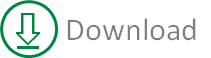Created August 2004
Last updated 5th August 2014

Microsoft® and Microsoft® Excel are registered trademarks of the Microsoft Corporation.
andypope.info is not associated with Microsoft. Copyright ©2007-2016 Andy Pope